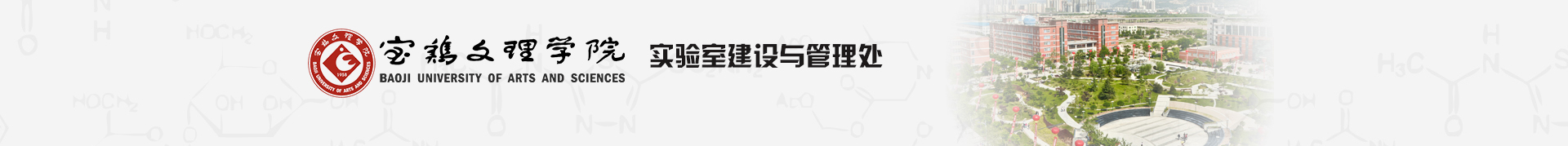﻿ 一楼-实验室管理处# 一楼

 1 2 3 4 5 6 7 8 9 10 11 12 13 14 15 16 17 18 19 20 21 22 23 24 25 26 27 28 29 30 31 32 33 34 35 36 37 38 39 40 41 42 43 44 45 46 实验楼 1 1099.9*13.2=130.68教务处多媒体教室 舞蹈室准备室 11013.2*13.2=174.24音乐系舞蹈室 11713.2*13.2=174.24音乐系舞蹈室 舞蹈室准备室 1189.9*13.2=130.68教务处多媒体教室 2 3 楼梯 4*7.9=31.6  值班室 4*4=16 1146.6*7.9=52.14教室管理科办公室 1156.6*7.9=52.14多媒体监控室 楼梯 4 5 1134*3.9=15.6 6 7 楼梯 楼梯 8 9 1089.9*13.2=130.68核心机房 1079.9*13.2=130.68音乐系舞蹈室 1209.9*13.2=130.68教务处多媒体教室 1199.9*13.2=130.68教务处多媒体教室 10 11 12 13 14 15 1063.48*4.2=14.616配电室 卫生间 一层 卫生间 1213.48*4.2=14.616配电室 16 17 1227.5*9.9=74.25教务处多媒体教室 18 1054.8*3.3=15.84实管处 1234.8*3.3=15.84设备处库房 19 20 1034.8*3.3=15.84实管处 1244.8*3.3=15.84设备处库房 21 22 101C美术学院烘烤室 楼梯 1257.5*9.9=74.25教务处多媒体教室 23 24 楼梯 25 大厅 大厅 26 101B7.5*3.6=27教育学院实验室 东 126A3*9=27舞蹈室准备室 27 28 101A9.9*16.2=160.38美术学院宝鸡民间美术展馆 126B9.9*16.2=160.38音乐系舞蹈室 29 30 31 32 33